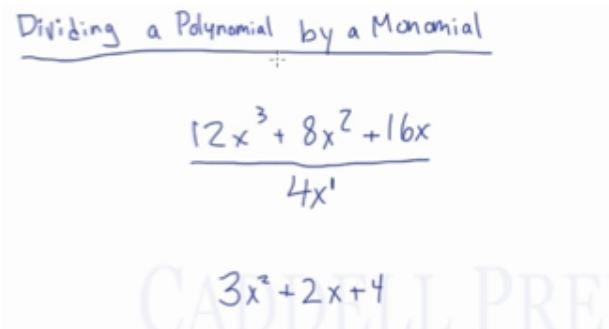In this video, learn how to divide a polynomial by a monomial. After you finish this lesson, view all of our Algebra 1 lessons and practice problems.

In this video, we are going to look at how to divide a polynomial by a monomial.

For example:
To solve:$(12x^3+8x^2+16x) \div 4x$
we will divide each term in the numerator separately by the denominator of 4x.
So:$12x^3 \div 4x=3x^2$$8x^2 \div 4x=2x$$16x \div 4x=4$$3x^2+2x+4$## Video-Lesson Transcript

In this short video, we’re going to review dividing a polynomial by a monomial.

For example:

We have a polynomial$12x^3 + 8x^2 + 16x$ divided by$4x$.

The very important point n dividing a polynomial by a monomial is you should divide each term of the numerator by the denominator.

Let’s go through this$\dfrac{12x^3 + 8x^2 + 16x}{4x^1}$$13x^2 + 2x + 4$

To recap, in dividing a polynomial by a monomial, we have to divide each term on the numerator by the denominator.

Each term must be able to be divided by the denominator in order to do so.